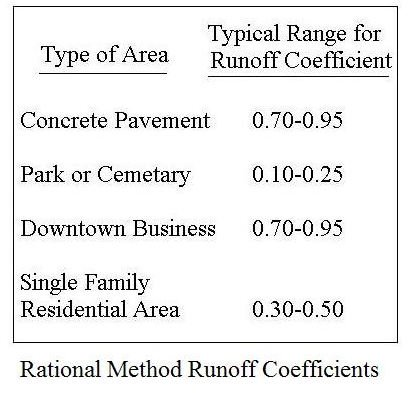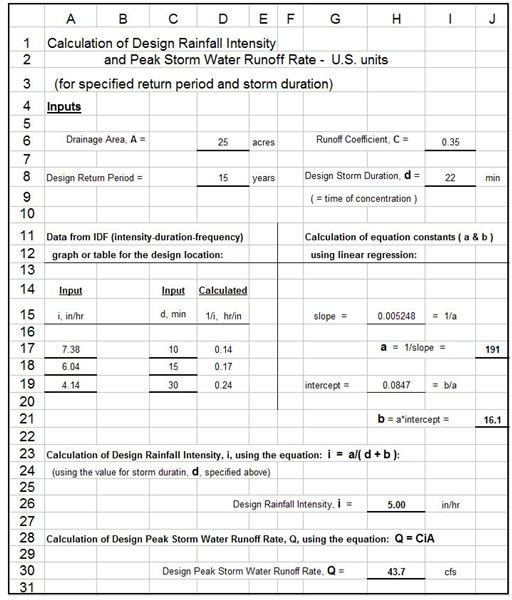# Use of Excel Formulas for Design Rainfall Intensity Calculation

## The Rational Method Equation and Parameters

The primary equation for Rational Method calculations is: Q = CiA, where:

• A is the area that drains to the design point of interest (the drainage area) in acres (for SI units: ha)
• C is the runoff coefficient for that drainage area, that is, the fraction of rainfall on the drainage area that becomes storm water runoff. It is dimensionless.
• i is the design rainfall intensity in inches/hr (for SI units: mm/hr)
• Q is the peak storm water runoff rate from the drainage area due to the design storm in cfs (for SI units: m3/s)

A note on units: The equation, Q = CiA is for the U.S. units given above. This equation actually gives Q in acre-in/hr. The unit conversion comes out to be 1.008 cfs/(acre-in/hr), so the equation, Q = 1.008CiA could be used, but in view of the accuracy with which the runoff coefficient can be determined, the conversion constant is typically taken to be simply 1. With the S.I. units given above for Q, i, and A, the Rational Method equation is Q = 0.0028 Cia.

Calculation of the peak storm water runoff rate, Q, is quite straightforward if values are known for C, i, and A.Some information on typical ranges for C values is given in the table at the right. The next section presents and discusses an Excel spreadsheet for calculating the design rainfall intensity, i, based on rainfall intensity duration frequency data for the design location, and then calculating the design storm water runoff rate, Q.

For more background information about the Rational Method and the parameters, Q, C, i, and A, see the article, "The Rational Method for Calculation of Peak Storm Water Runoff Rate." For background information about calculating design rainfall intensity, i, see the article, "Calculating Design Rainfall Intensity for Use in the Rational Method."

## Excel Formulas for Design Rainfall Intensity and Rational Method CalculationsThe Excel spreadsheet image shown at the left is set up with Excel formulas to derive an equation for calculating the design rainfall intensity, i. The Excel formulas also calculate i, and then use the Rational Method equation to calculate the design peak storm water runoff rate, Q. The input information needed is: i) the drainage area, A in acres; ii) the runoff coefficient of the drainage area, C; iii) the design return period in years; and iv) the design storm duration, d, in minutes. In the spreadsheet that can be downloaded through the link below, there’s a table with ranges of values of the runoff coefficient, C, for several types of land use.

There is also provision in the spreadsheet for entering data points for storm intensity (in/hr) vs storm duration (min) for the specified design return period. This data must come from a rainfall intensity duration frequency graph or table for the design location. In the U.S. this information is typically available in each state from some state agency. Many are available on state agency websites.

The Excel formulas in the spreadsheet are set up to calculate the constants, a and b, in the equation i = a/( d + b ), by linear regression of 1/i vs d. Then the design rainfall intensity is calculated with the equation i = a/( d + b ) using the calculated values for a and b. Finally the design peak storm water runoff rate is calculated using the Rational Method equation, Q = CiA. This spreadsheet can be downloaded for U.S. units or for S.I. units by clicking on the appropriate link below.

## References

References for Further Information:

1. Bengtson, Harlan H., Hydraulic Design of Storm Sewers, Including the Use of Excel, an online, continuing education course for PDH credit.

2. McCuen, Richard H., Hydrologic Analysis and Design, 2nd Ed, Upper Saddle River, NJ, 1998.

3. Knox County Tennessee, Stormwater Management Manual, section on the Rational Method

## This post is part of the series: The Rational Method for Calculating Peak Storm Water Runoff Rate

The Rational Method is widely used to calculate the peak storm water runoff rate for a variety of storm water management applications. The drainage area, runoff coefficient, and design rainfall intensity are needed for the storm water runoff calculation.